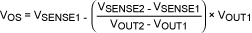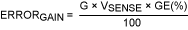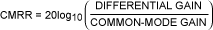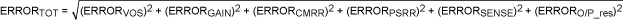# 直流误差计算器简化检流放大器的优化

### Abstract

This article describes a systematic approach for calculating the total DC error budget of a current-sense amplifier (CSA). The individual sources of error are discussed and a method for estimating the total error budget is presented. Finally, a step-by-step process explains how to use calculator software, developed to help quickly compute the total error of the selected CSA.

### 输入失调电压(式1)
CSA输入失调电压产生的输出失调误差如下：
 ERRORVOS = G × VOS (式2)

### 增益误差(式3)(式4)(式5)

### 共模抑制比(式6)

 ERRORCMRR = G × Maximum [Abs Value (Min VCM - Data Sheet VCM), Abs Value (Max VCM - Data Sheet VCM)] × 10-CMRR/20 (式7)

### 电源抑制比

 ERRORPSRR = G × Maximum [Abs Value (Min VDD - Data Sheet VDD), Abs Value (Max VDD - Data Sheet VDD)] × 10-PSRR/20 (式8)

### 检流电阻误差(式9)

### 输出电阻误差(式10)

### 估算系统误差(式11)(式12)

### 检流误差估算计算器的使用

1. 输入触发点 = 50A (单向)
2. 检流电阻误差 = 0.5%
3. 检流电阻 = < 1mΩ
4. 电源电压范围 = 4.5V - 5.5V
5. 输入共模电压范围 = 12V - 18V
6. 总误差预算 = < 2%，这意味着CSA增益误差< 2%，失调误差VOS < 1mV，因为每项误差不能超过总误差。

#### 第二步、检流误差计算器输入#### 第三步、验证数据手册规格#### 第五步、选择不同的CSA

 Table 1. Total Error Budget Computed Using the Calculator Part Total Error (%) Conditions T = 25°C T = -40°C to +85°C MAX9922 0.64 0.79 Gain = 60 MAX9918 1.13 1.93 Gain = 60 MAX9929F 1.39 3.45 — MAX4080S 1.43 2.55 — MAX4373F 2.84 6.18 — MAX4172 — 2.57 O/P termination = 6kΩ